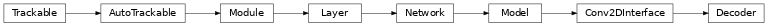Decoder¶

Inheritance Diagramclass ashpy.models.convolutional.decoders.Decoder(layer_spec_input_res, layer_spec_target_res, kernel_size, initial_filters, filters_cap, channels, use_dropout=True, dropout_prob=0.3, non_linearity=<class 'tensorflow.python.keras.layers.advanced_activations.LeakyReLU'>)[source]

Primitive Model for all decoder (i.e., transpose convolution) based architecture.

Notes

Default to DCGAN Generator architecture.

Examples

• Direct Usage:

dummy_generator = Decoder(
layer_spec_input_res=(8, 8),
layer_spec_target_res=(64, 64),
kernel_size=(5, 5),
initial_filters=1024,
filters_cap=16,
channels=3,
)

• Subclassing

class DummyGenerator(Decoder):
def call(self, input, training=True):
print("Dummy Generator!")
return input

dummy_generator = DummyGenerator(
layer_spec_input_res=(8, 8),
layer_spec_target_res=(32, 32),
kernel_size=(5, 5),
initial_filters=1024,
filters_cap=16,
channels=3,
)
dummy_generator(tf.random.normal((1, 100)))

Dummy Generator!

Methods

 __init__(layer_spec_input_res, …[, …]) Instantiate the Decoder.

Attributes

 activity_regularizer Optional regularizer function for the output of this layer. dtype dynamic inbound_nodes Deprecated, do NOT use! Only for compatibility with external Keras. input Retrieves the input tensor(s) of a layer. input_mask Retrieves the input mask tensor(s) of a layer. input_shape Retrieves the input shape(s) of a layer. input_spec Gets the network’s input specs. layers losses Losses which are associated with this Layer. metrics Returns the model’s metrics added using compile, add_metric APIs. metrics_names Returns the model’s display labels for all outputs. name Returns the name of this module as passed or determined in the ctor. name_scope Returns a tf.name_scope instance for this class. non_trainable_variables non_trainable_weights outbound_nodes Deprecated, do NOT use! Only for compatibility with external Keras. output Retrieves the output tensor(s) of a layer. output_mask Retrieves the output mask tensor(s) of a layer. output_shape Retrieves the output shape(s) of a layer. run_eagerly Settable attribute indicating whether the model should run eagerly. sample_weights state_updates Returns the updates from all layers that are stateful. stateful submodules Sequence of all sub-modules. trainable trainable_variables Sequence of variables owned by this module and it’s submodules. trainable_weights updates variables Returns the list of all layer variables/weights. weights Returns the list of all layer variables/weights.
__init__(layer_spec_input_res, layer_spec_target_res, kernel_size, initial_filters, filters_cap, channels, use_dropout=True, dropout_prob=0.3, non_linearity=<class 'tensorflow.python.keras.layers.advanced_activations.LeakyReLU'>)[source]

Instantiate the Decoder.

Model Assembly:

2. _add_building_block(): Core of the model, the layers specified here get added to the tf.keras.Model multiple times consuming the hyperparameters generated in the _get_layer_spec().

3. _add_final_block(): Final block of our tf.keras.Model, take the model after _add_building_block() and prepare them for the for the final output.

Parameters
• layer_spec_input_res (tuple of (int, int)) – Shape of the _get_layer_spec() input tensors.

• layer_spec_target_res – (tuple of (int, int)): Shape of tensor desired as output of _get_layer_spec().

• kernel_size (tuple of (int, int)) – Kernel used by the convolution layers.

• initial_filters (int) – Numbers of filters at the end of the first block.

• filters_cap (int) – Cap filters to a set amount, in the case of Decoder is a floor value AKA the minimum amount of filters.

• channels (int) – Channels of the output images (1 for Grayscale, 3 for RGB).

Returns

None

Raises

ValueError – If filters_cap > initial_filters.

Construct the core of the tf.keras.Model.

The layers specified here get added to the tf.keras.Model multiple times consuming the hyperparameters generated in the _get_layer_spec().

Parameters

filters (int) – Number of filters to use for this iteration of the Building Block.

Prepare results of _add_building_block() for the for the final output.

Parameters

channels (int) – Channels of the output images (1 for Grayscale, 3 for RGB).